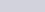ChemTalk

# Charles’ Law## Core Concepts

In this article, you will learn how temperature relates to volume, and how to use Charles’ law formula to solve problems regarding the change in temperature and volume of a system.

## Important Things to Consider

The gas law described in this article only applies to ideal gases, which you can read about on our article, The Ideal Gas Law. Non-ideal gases, under conditions of high pressures or low temperatures, do not follow Charles’ Law due to the effects of attractive and repulsive forces between gas particles.

## Increasing Temperature

Consider a piston containing 1 liter of gas. Because the piston is free to change volume, the pressure remains constant.

Now, consider what happens when we increase the temperature of the gas. From Kinetic Molecular Theory, we know that the temperature of a sample of gas is directly related to its velocity.

From our article on pressure, What is Pressure, you know that the pressure of a gas is a result of force exerted when colliding with a wall. This force, as stated in the article on pressure, can be calculated with the equation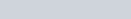.

As temperature increases, the value of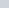increases, and therefore the force increases. However, because the piston is free to change volume, the pressure remains constant. From Boyle’s Law, we know that pressure is inversely proportional to volume, and thus the piston would increase its volume to account for the change in temperature.

## Charles’ Law

French physicist Jacques Charles also noticed this phenomenon, and discovered the relation between temperature and volume. This is what is known as Charles’ law or Charles’ law formula, and can be written as: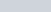. It can also be expressed as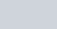, where variables markedare the initial conditions, and variables marked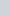are the final ones.

Charles discovered this relationship between temperature and volume through experiments involving balloons. Specifically, he filled five balloons with equal volumes of different gases. Then, he raised the temperature of the balloons and observed that each increased in volume the same amount. For this, Charles is creditted with first suggesting empirically that volume and temperature have a direct proportional relationship in 1787. However, this relationship would not be dubbed “Charles’ Law” until 1802, when another French physicist, Joseph-Louis Guy-Lussac produced more convincing evidence for the law.

## Applications

Because of this proportionality, we can use Charles’ law to see how a system would react to a change in temperature, or how a system would react to a change in volume (both with constant pressure). As temperature increases, volume increases, and vice versa.

## Charles’ Law Worked Examples

Here’s how you would solve an example Charles’ law problem.

Problem: Calculate the change in temperature when a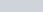piston of oxygen gas at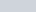is compressed to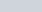.Here’s another Charles’ law problem.

Problem: What change in volume results if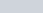of oxygen is cooled by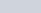from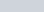?## Charles’ Law Practice Problems

Problem 1

You have aballoon full of air at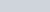. The temperature at which air becomes plasma is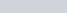. Assuming the material of the balloon is completely unbreakable and resistant to high temperatures, what is the maximum volume that you could expand the air in the balloon by heating before the air turns to plasma?

Problem 2

You have an aquarium of ice water at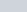with the dimensions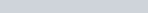and a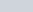balloon filled with room temperature,air. You submerge the balloon in the aquarium, cooling the air inside to. How much does the water level of the aquarium rise from before you submerged the balloon to when the balloon completely cools to? (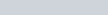)

## Charles’ Law Practice Problem Solutions

1: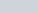2: